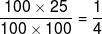Courses

# Mathematics Test 4 - Matrices And Determinants, Probability, Indefinite Integration

## 25 Questions MCQ Test Mock Test Series for JEE Main & Advanced 2022 | Mathematics Test 4 - Matrices And Determinants, Probability, Indefinite Integration

Description
This mock test of Mathematics Test 4 - Matrices And Determinants, Probability, Indefinite Integration for JEE helps you for every JEE entrance exam. This contains 25 Multiple Choice Questions for JEE Mathematics Test 4 - Matrices And Determinants, Probability, Indefinite Integration (mcq) to study with solutions a complete question bank. The solved questions answers in this Mathematics Test 4 - Matrices And Determinants, Probability, Indefinite Integration quiz give you a good mix of easy questions and tough questions. JEE students definitely take this Mathematics Test 4 - Matrices And Determinants, Probability, Indefinite Integration exercise for a better result in the exam. You can find other Mathematics Test 4 - Matrices And Determinants, Probability, Indefinite Integration extra questions, long questions & short questions for JEE on EduRev as well by searching above.
QUESTION: 1

### If  n ≠ 3k and 1 ,ω,ω2 are the cube roots of unity then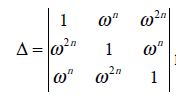has the value

Solution:

Del=(1-w^3n)+w^n(0)+w^2n(w^4n-w^n) =1-(w^3)^n+(w^6)^n-(w^3)^n =1-1+1-1 =0

QUESTION: 2

Solution:
QUESTION: 3

###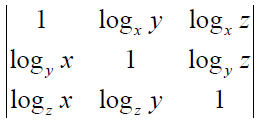x,y,z being +ive equals

Solution:
QUESTION: 4
The roots of the equation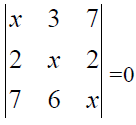are

Solution:
QUESTION: 5
If the three equations are consistent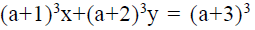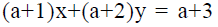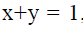, then a =
Solution:
QUESTION: 6

The number of values of k for which the system of equations ( k+1)x + 8y = 4k, kx + (k+3)y = 3k - 1 has inifinitely many solutions is

Solution:

For infinitely many solutions the two equation become identical.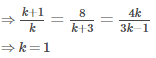QUESTION: 7
If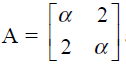and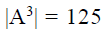, then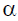is
Solution:
QUESTION: 8

Let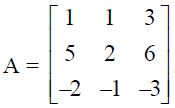. Then A is

Solution:
QUESTION: 9

IF B is a non-singular matrix and A is a square matrix, then  det (B-1 AB)

Solution:
QUESTION: 10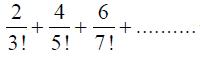will be equal to

Solution:
QUESTION: 11
The sum of the series is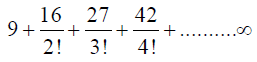Solution:
QUESTION: 12
The sum of the series is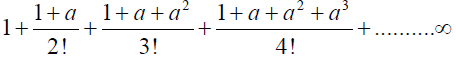Solution:
QUESTION: 13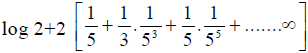is equal to
Solution:
QUESTION: 14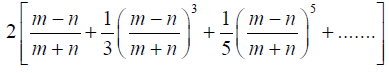is equal to
Solution:
QUESTION: 15

Two cards are drawn at random and without replacement from a pack of 52 playing cards. Find the probability that both the cards are black.

Solution:
QUESTION: 16

Seven chits are numbered 1 to 7. Three are drawn one by one with replacements. The probability that the least number on any selected chit is 5, is

Solution:
QUESTION: 17

A student appear for tests I,II and III. The student is successful if he passes either in tests I and II or tests I and III. The probabilities of the student passing in tests I,II and III are p,q and 1/2 respectively. If the probability that the student is successful is 1/2, then

Solution:
QUESTION: 18

Words from the letters of the word PROBABILITY are formed by talking all at a time.The probability that both B’s are together & both I’s are together is

Solution:
QUESTION: 19

The probability that a person will hit a target in a shooting practice is 0.3. If the shoots 10 times, the probability that he hit the target is

Solution:
QUESTION: 20

The probability that Krishna will be alive 10 years hence is 7/15 and that Hari will be alive is 7/10. What is the probability that both Krishna and Hari will be dead 10 years hence

Solution:
*Answer can only contain numeric values
QUESTION: 21

Value of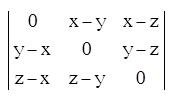is

Solution:

Value of odd order skew symmetric determinant

*Answer can only contain numeric values
QUESTION: 22

If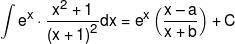then value of (a + b) = ?

Solution: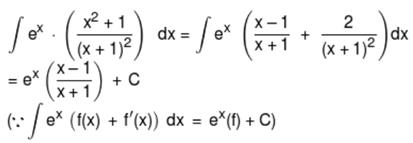*Answer can only contain numeric values
QUESTION: 23

Three children are selected at random from a group of 6 boys and 4 girls. It is known that in this group exactly one girl and one boy belong to same parents. The probability that the selected group of children have no blood relations, is equal to?

Solution:

P(boy and his sister both are selected)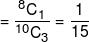∴  Required probability
= 1 – 1/15 = 14/15 = 0.93

*Answer can only contain numeric values
QUESTION: 24

The probability that the 13th day of a randomly chosen month is a Friday, is?

Solution:

Probability of selecting a month = 1/12.
13th day of a randomly selected month is Friday if its first day is Sunday.
Probability of this event = 1/7.
Hence required probability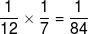= 0.01

*Answer can only contain numeric values
QUESTION: 25

If two integers m and n are chosen at random from 1 to 100 then the probability that a number of the form 7m + 7n is divisible by 5 equals:

Solution:

Since m and n are selected between 1 and 100, hence sample space = 100 × 100
Also, 71 = 7,  72 = 49, 73 = 343, 74 = 2401,
75 = 16807 etc. Hence 1, 3, 7 and 9 will be the last digits in the powers of 7.
n, m →

1,1       1,2       1,3 ...........       1, 100
2,1       2,2       2,3 ...........       2, 100
...........................................
100, 1  100,2   100, 3.........     100, 100
For m = 1 ; n = 3, 7, 11, ...... 97
∴ Favourable cases =  25
For m = 2, n = 4, 8, 12, ......., 100
∴  Favourable cases =  25
Similarly for every m, favourable n are 25.
∴  Total favourable cases  = 100 × 25
Hence required probability =